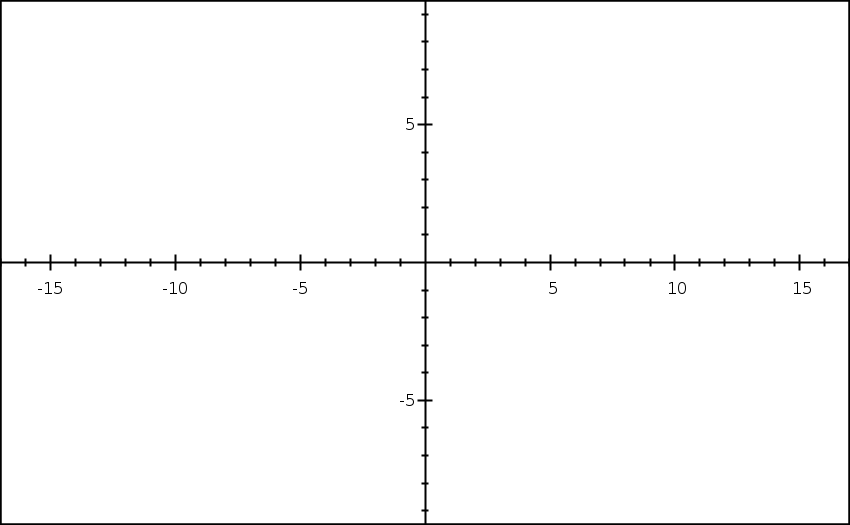Search IntMath
Close

450+ Math Lessons written by Math Professors and Teachers

5 Million+ Students Helped Each Year

1200+ Articles Written by Math Educators and Enthusiasts

Simplifying and Teaching Math for Over 23 Years

# Friday math movie - Limits

By Murray Bourne, 30 Jan 2009

Students always seem to struggle with the concept of limits.

Most introductions to calculus include a section on limits (as they should), but because of the difficulty, students end up having negative reactions about calculus before they get on to the "real" stuff of differentiation and integration. Nevertheless, limits are a very important concept in calculus.

Here are a few movies that introduce what limits are about. They are highly rated on YouTube and hopefully they’ll help you to figure out the concepts.

## Introduction to Limits Part 1

This is the first in a series. You'll see links to the rest when the movie finishes.

## What is a Limit? Basic Idea of Limits

This guy points out one of the stumbling blocks — the notation for limits can be quite confusing.

## Limits at Infinity - Basic Idea and Shortcuts

This one talks about limits "at" infinity. But of course, we can only talk about limits as x approaches infinity.

Anyway, don't sweat about limits and continuity at first. Come back to it after you have been doing differentiation and integration for a while and it will hopefully make more sense. At least by then you will know how limits get used.

### 4 Comments on “Friday math movie - Limits”

1. Hisni says:

2. pierre-richard villedrouin says:

Graphs in book are reduced sensorial vision.With the video animation the graph becomes a track, brain cells expect at the Limit the OMINOUS encounter.The screen becomes a stage.

3. gagangc says:

Sir,
what is limit(n->infinity)(1+(1/n))^n

According to me as n tends to infinity 1/n tends to 0.so (1+(1/n)) tends to 1.and 1^infinity is always 1.

But my text book says answer is e. why?

4. Murray says:

@gagangc: Ah, this is one of the many wonderful surprises in math.

If you try some large numbers (like n = 1000, or n = 100000), you will see the value does indeed approach e = 2.71828...

This graph shows the values for n > 0. The red line is at "e".We actually define that limit to be equal to "e". See this article on Dr Math for some more background.

### Comment Preview

HTML: You can use simple tags like <b>, <a href="...">, etc.

To enter math, you can can either:

1. Use simple calculator-like input in the following format (surround your math in backticks, or qq on tablet or phone):
a^2 = sqrt(b^2 + c^2)
(See more on ASCIIMath syntax); or
2. Use simple LaTeX in the following format. Surround your math with $$ and $$.
$$\int g dx = \sqrt{\frac{a}{b}}$$
(This is standard simple LaTeX.)

NOTE: You can mix both types of math entry in your comment.